# Selina Solutions Concise Mathematics Class 6 Chapter 34 Mean and Median

Selina Solutions Concise Mathematics Class 6 Chapter 34 Mean and Median has answers in a descriptive manner, prepared by subject experts at BYJU’S. Students improve their analytical and logical thinking abilities, using these solutions. Those who aspire to speed up their problem solving skills are suggested to practice these solutions on a regular basis. Here, students can download Selina Solutions Concise Mathematics Class 6 Chapter 34 Mean and Median PDF, from the links which are given below.

Chapter 34 describes finding the mean and median for a given set of numbers, with examples. Students can cross check their answers while revising the textbook questions, referring to solutions which are provided in PDF format.

## Selina Solutions Concise Mathematics Class 6 Chapter 34 Mean and Median Download PDF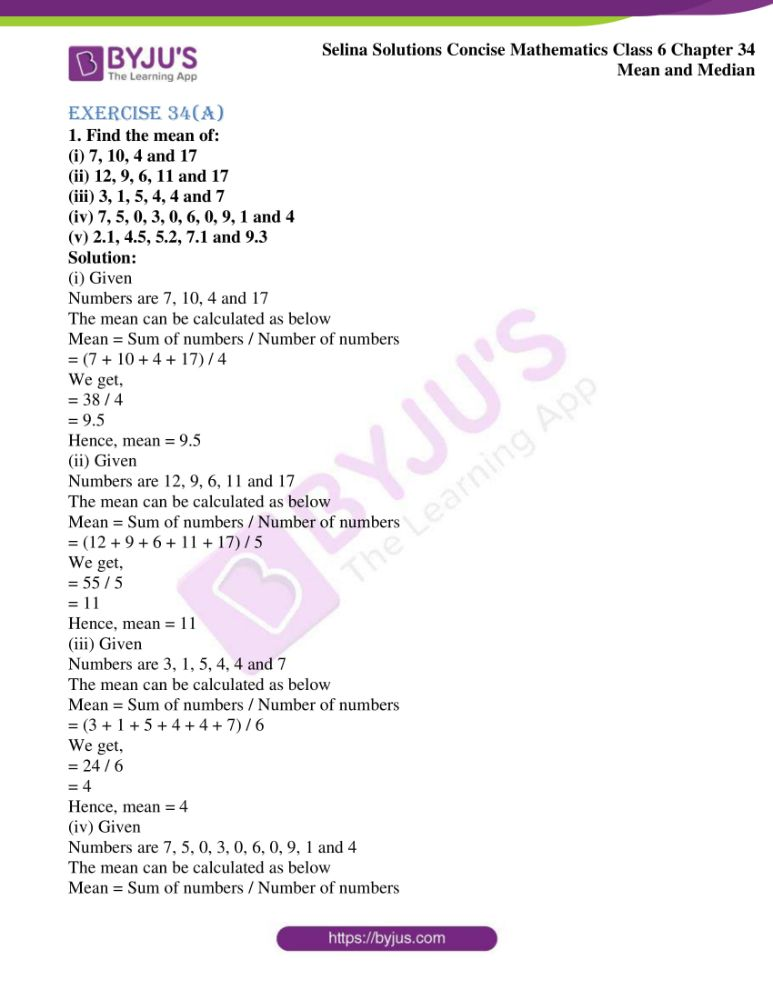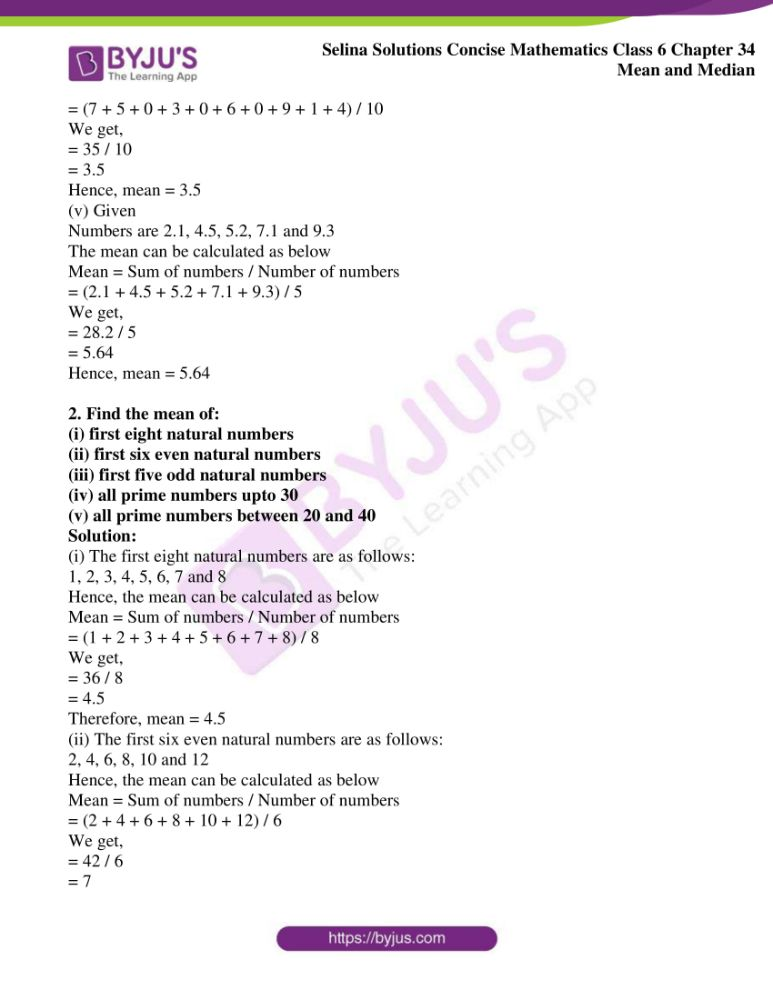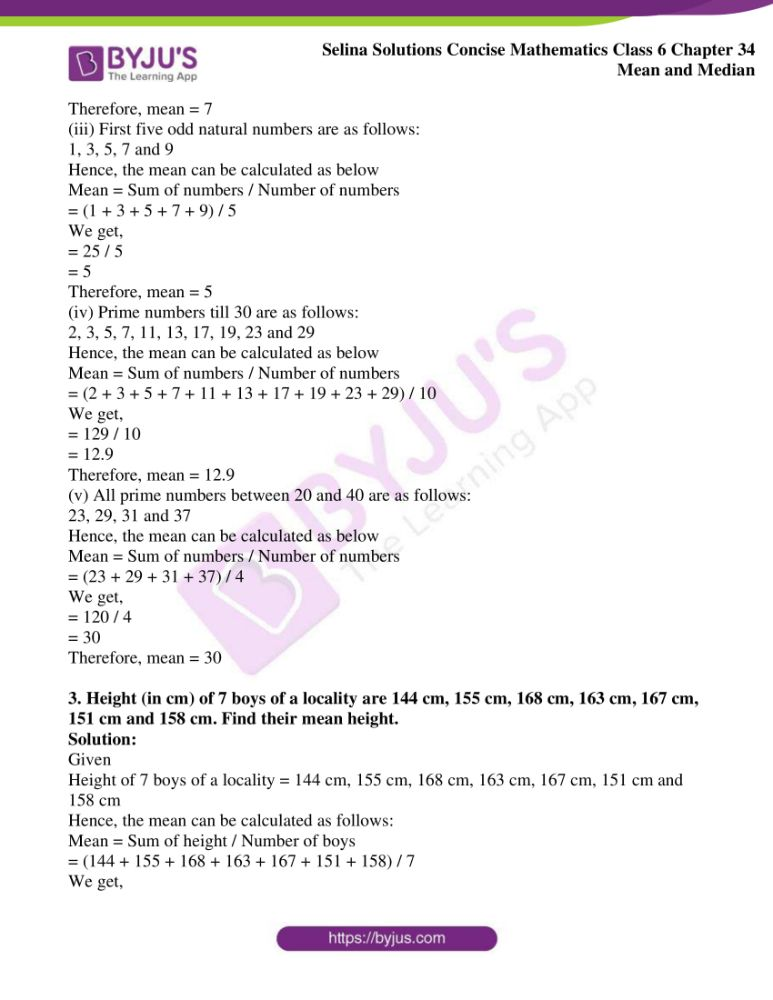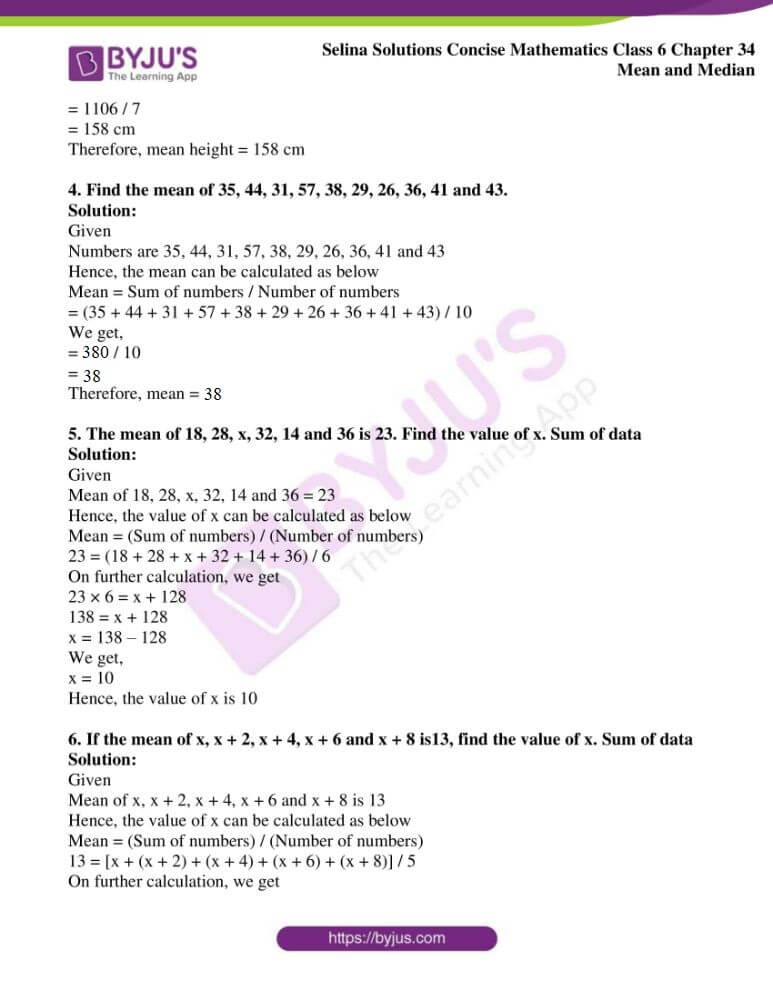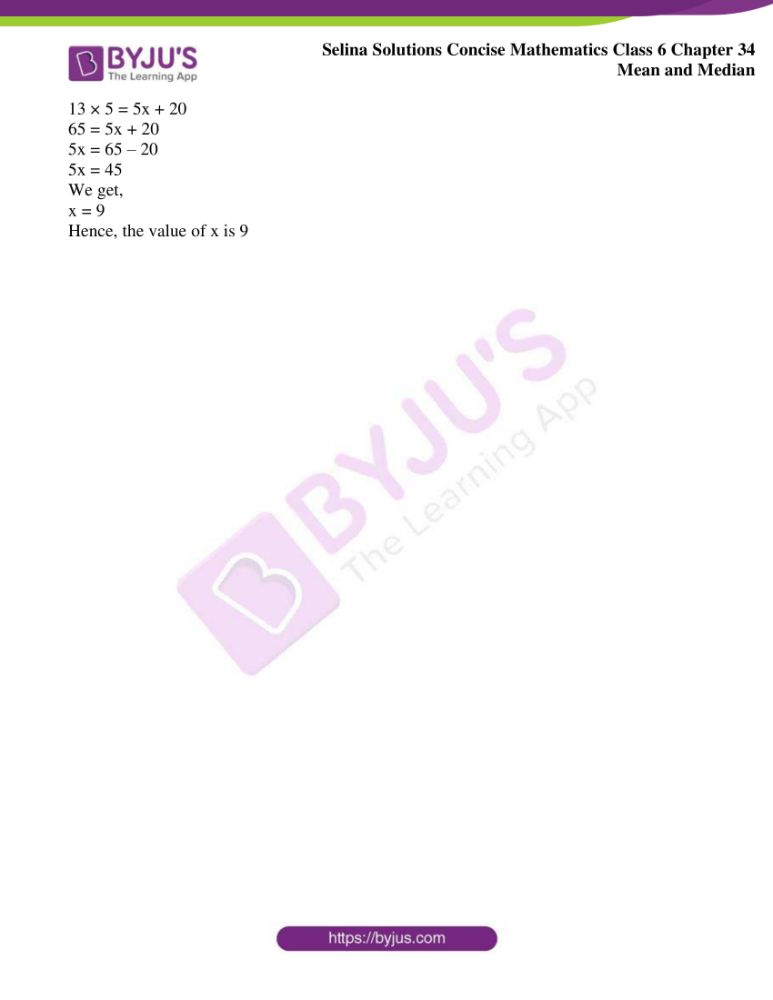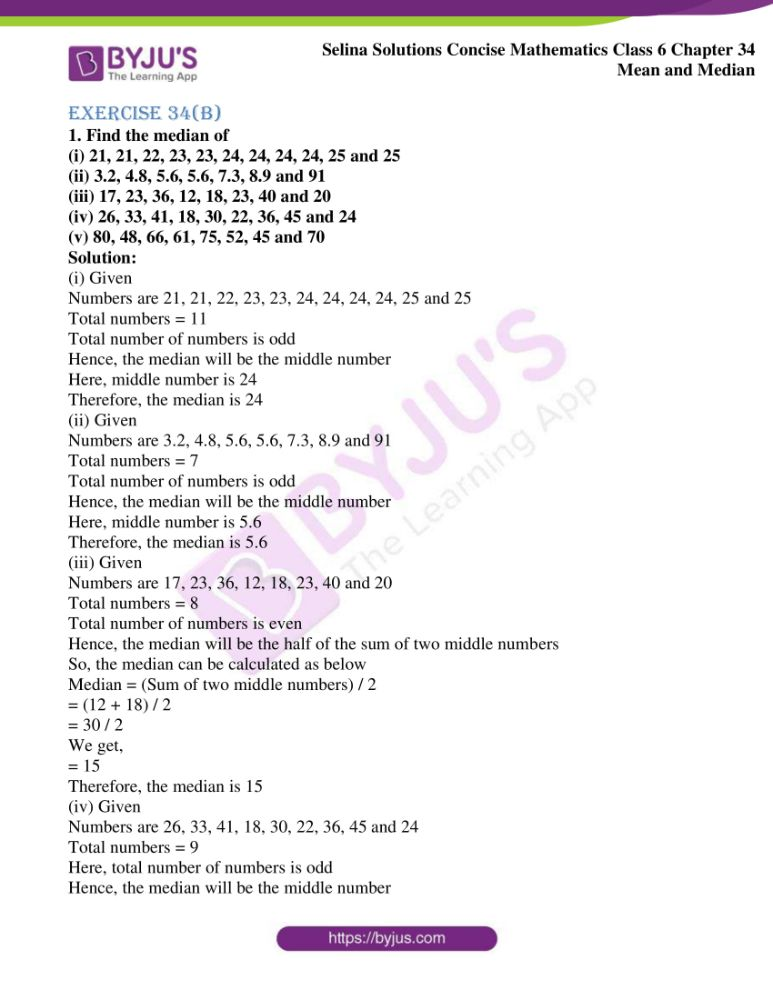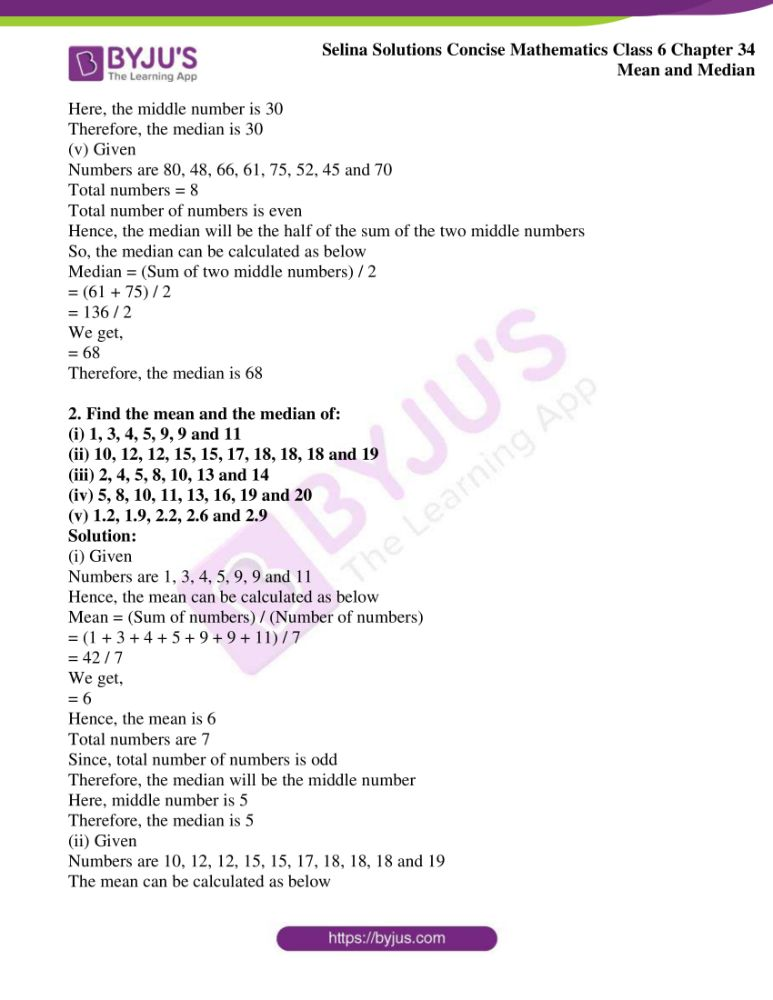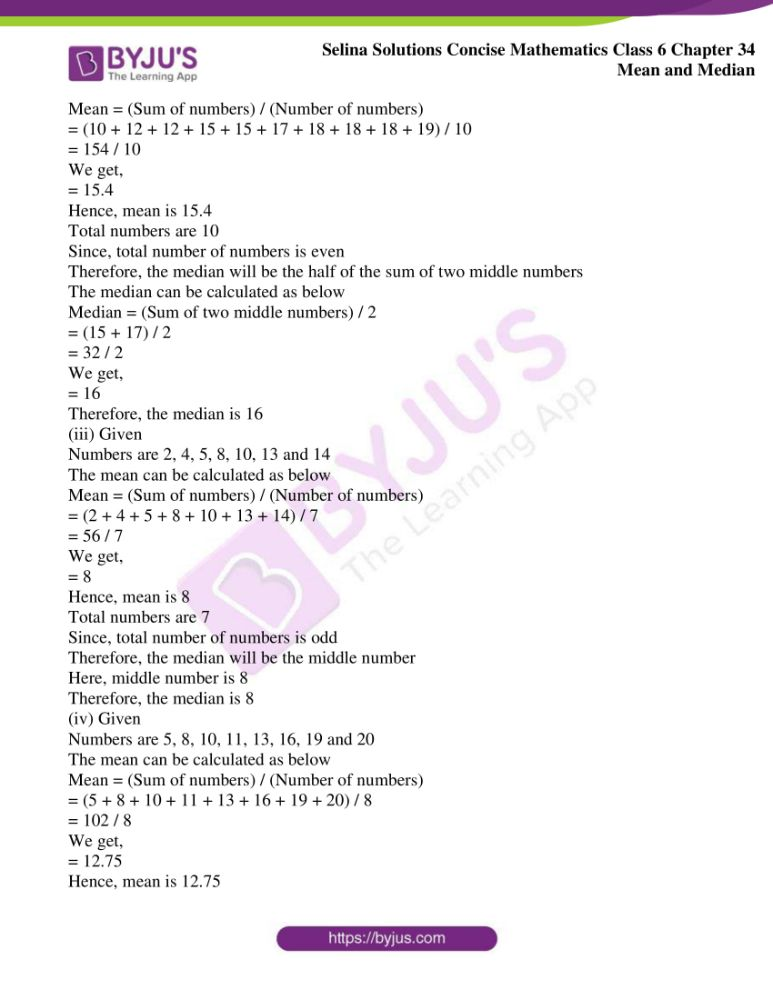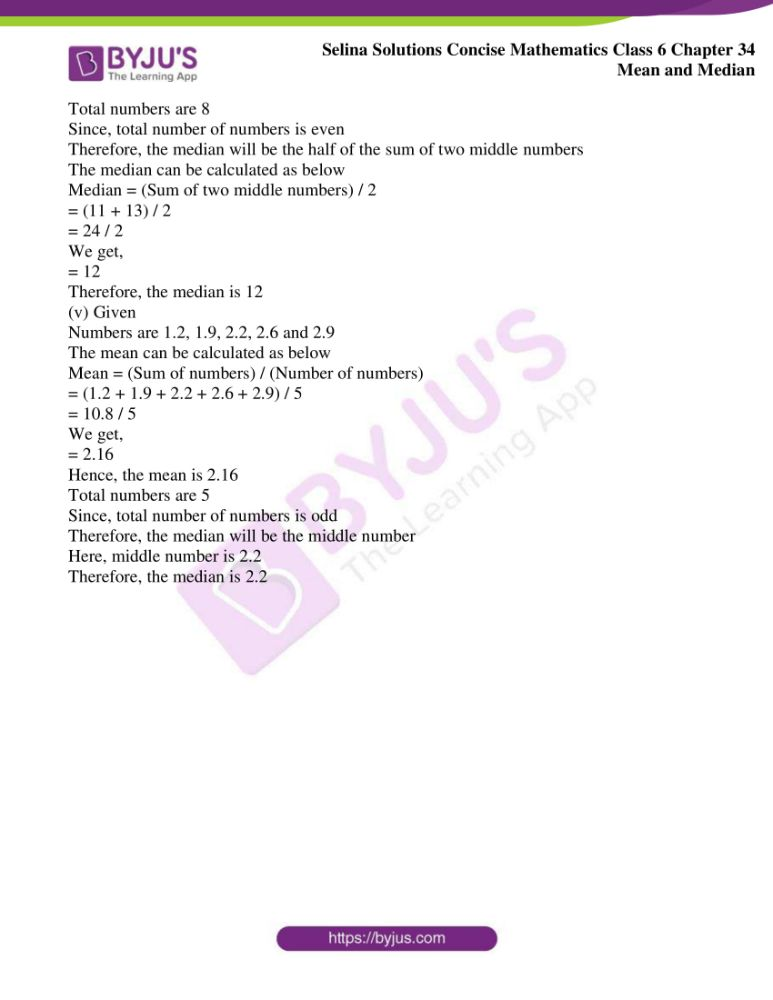### Exercises of Selina Solutions Concise Mathematics Class 6 Chapter 34 Mean and Median

Exercise 34(A) Solutions

Exercise 34(B) Solutions

## Access Selina Solutions Concise Mathematics Class 6 Chapter 34 Mean and Median

Exercise 34(A)

1. Find the mean of:

(i) 7, 10, 4 and 17

(ii) 12, 9, 6, 11 and 17

(iii) 3, 1, 5, 4, 4 and 7

(iv) 7, 5, 0, 3, 0, 6, 0, 9, 1 and 4

(v) 2.1, 4.5, 5.2, 7.1 and 9.3

Solution:

(i) Given

Numbers are 7, 10, 4 and 17

The mean can be calculated as below

Mean = Sum of numbers / Number of numbers

= (7 + 10 + 4 + 17) / 4

We get,

= 38 / 4

= 9.5

Hence, mean = 9.5

(ii) Given

Numbers are 12, 9, 6, 11 and 17

The mean can be calculated as below

Mean = Sum of numbers / Number of numbers

= (12 + 9 + 6 + 11 + 17) / 5

We get,

= 55 / 5

= 11

Hence, mean = 11

(iii) Given

Numbers are 3, 1, 5, 4, 4 and 7

The mean can be calculated as below

Mean = Sum of numbers / Number of numbers

= (3 + 1 + 5 + 4 + 4 + 7) / 6

We get,

= 24 / 6

= 4

Hence, mean = 4

(iv) Given

Numbers are 7, 5, 0, 3, 0, 6, 0, 9, 1 and 4

The mean can be calculated as below

Mean = Sum of numbers / Number of numbers

= (7 + 5 + 0 + 3 + 0 + 6 + 0 + 9 + 1 + 4) / 10

We get,

= 35 / 10

= 3.5

Hence, mean = 3.5

(v) Given

Numbers are 2.1, 4.5, 5.2, 7.1 and 9.3

The mean can be calculated as below

Mean = Sum of numbers / Number of numbers

= (2.1 + 4.5 + 5.2 + 7.1 + 9.3) / 5

We get,

= 28.2 / 5

= 5.64

Hence, mean = 5.64

2. Find the mean of:

(i) first eight natural numbers

(ii) first six even natural numbers

(iii) first five odd natural numbers

(iv) all prime numbers upto 30

(v) all prime numbers between 20 and 40

Solution:

(i) The first eight natural numbers are as follows:

1, 2, 3, 4, 5, 6, 7 and 8

Hence, the mean can be calculated as below

Mean = Sum of numbers / Number of numbers

= (1 + 2 + 3 + 4 + 5 + 6 + 7 + 8) / 8

We get,

= 36 / 8

= 4.5

Therefore, mean = 4.5

(ii) The first six even natural numbers are as follows:

2, 4, 6, 8, 10 and 12

Hence, the mean can be calculated as below

Mean = Sum of numbers / Number of numbers

= (2 + 4 + 6 + 8 + 10 + 12) / 6

We get,

= 42 / 6

= 7

Therefore, mean = 7

(iii) First five odd natural numbers are as follows:

1, 3, 5, 7 and 9

Hence, the mean can be calculated as below

Mean = Sum of numbers / Number of numbers

= (1 + 3 + 5 + 7 + 9) / 5

We get,

= 25 / 5

= 5

Therefore, mean = 5

(iv) Prime numbers till 30 are as follows:

2, 3, 5, 7, 11, 13, 17, 19, 23 and 29

Hence, the mean can be calculated as below

Mean = Sum of numbers / Number of numbers

= (2 + 3 + 5 + 7 + 11 + 13 + 17 + 19 + 23 + 29) / 10

We get,

= 129 / 10

= 12.9

Therefore, mean = 12.9

(v) All prime numbers between 20 and 40 are as follows:

23, 29, 31 and 37

Hence, the mean can be calculated as below

Mean = Sum of numbers / Number of numbers

= (23 + 29 + 31 + 37) / 4

We get,

= 120 / 4

= 30

Therefore, mean = 30

3. Height (in cm) of 7 boys of a locality are 144 cm, 155 cm, 168 cm, 163 cm, 167 cm, 151 cm and 158 cm. Find their mean height.

Solution:

Given

Height of 7 boys of a locality = 144 cm, 155 cm, 168 cm, 163 cm, 167 cm, 151 cm and 158 cm

Hence, the mean can be calculated as follows:

Mean = Sum of height / Number of boys

= (144 + 155 + 168 + 163 + 167 + 151 + 158) / 7

We get,

= 1106 / 7

= 158 cm

Therefore, mean height = 158 cm

4. Find the mean of 35, 44, 31, 57, 38, 29, 26, 36, 41 and 43.

Solution:

Given

Numbers are 35, 44, 31, 57, 38, 29, 26, 36, 41 and 43

Hence, the mean can be calculated as below

Mean = Sum of numbers / Number of numbers

= (35 + 44 + 31 + 57 + 38 + 29 + 26 + 36 + 41 + 43) / 10

We get,

= 380 / 10

= 38

Therefore, mean = 38

5. The mean of 18, 28, x, 32, 14 and 36 is 23. Find the value of x. Sum of data

Solution:

Given

Mean of 18, 28, x, 32, 14 and 36 = 23

Hence, the value of x can be calculated as below

Mean = (Sum of numbers) / (Number of numbers)

23 = (18 + 28 + x + 32 + 14 + 36) / 6

On further calculation, we get

23 × 6 = x + 128

138 = x + 128

x = 138 – 128

We get,

x = 10

Hence, the value of x is 10

6. If the mean of x, x + 2, x + 4, x + 6 and x + 8 is13, find the value of x. Sum of data

Solution:

Given

Mean of x, x + 2, x + 4, x + 6 and x + 8 is 13

Hence, the value of x can be calculated as below

Mean = (Sum of numbers) / (Number of numbers)

13 = [x + (x + 2) + (x + 4) + (x + 6) + (x + 8)] / 5

On further calculation, we get

13 × 5 = 5x + 20

65 = 5x + 20

5x = 65 – 20

5x = 45

We get,

x = 9

Hence, the value of x is 9

Exercise 34(B)

1. Find the median of

(i) 21, 21, 22, 23, 23, 24, 24, 24, 24, 25 and 25

(ii) 3.2, 4.8, 5.6, 5.6, 7.3, 8.9 and 91

(iii) 17, 23, 36, 12, 18, 23, 40 and 20

(iv) 26, 33, 41, 18, 30, 22, 36, 45 and 24

(v) 80, 48, 66, 61, 75, 52, 45 and 70

Solution:

(i) Given

Numbers are 21, 21, 22, 23, 23, 24, 24, 24, 24, 25 and 25

Total numbers = 11

Total number of numbers is odd

Hence, the median will be the middle number

Here, middle number is 24

Therefore, the median is 24

(ii) Given

Numbers are 3.2, 4.8, 5.6, 5.6, 7.3, 8.9 and 91

Total numbers = 7

Total number of numbers is odd

Hence, the median will be the middle number

Here, middle number is 5.6

Therefore, the median is 5.6

(iii) Given

Numbers are 17, 23, 36, 12, 18, 23, 40 and 20

Total numbers = 8

Total number of numbers is even

Hence, the median will be the half of the sum of two middle numbers

So, the median can be calculated as below

Median = (Sum of two middle numbers) / 2

= (12 + 18) / 2

= 30 / 2

We get,

= 15

Therefore, the median is 15

(iv) Given

Numbers are 26, 33, 41, 18, 30, 22, 36, 45 and 24

Total numbers = 9

Here, total number of numbers is odd

Hence, the median will be the middle number

Here, the middle number is 30

Therefore, the median is 30

(v) Given

Numbers are 80, 48, 66, 61, 75, 52, 45 and 70

Total numbers = 8

Total number of numbers is even

Hence, the median will be the half of the sum of the two middle numbers

So, the median can be calculated as below

Median = (Sum of two middle numbers) / 2

= (61 + 75) / 2

= 136 / 2

We get,

= 68

Therefore, the median is 68

2. Find the mean and the median of:

(i) 1, 3, 4, 5, 9, 9 and 11

(ii) 10, 12, 12, 15, 15, 17, 18, 18, 18 and 19

(iii) 2, 4, 5, 8, 10, 13 and 14

(iv) 5, 8, 10, 11, 13, 16, 19 and 20

(v) 1.2, 1.9, 2.2, 2.6 and 2.9

Solution:

(i) Given

Numbers are 1, 3, 4, 5, 9, 9 and 11

Hence, the mean can be calculated as below

Mean = (Sum of numbers) / (Number of numbers)

= (1 + 3 + 4 + 5 + 9 + 9 + 11) / 7

= 42 / 7

We get,

= 6

Hence, the mean is 6

Total numbers are 7

Since, total number of numbers is odd

Therefore, the median will be the middle number

Here, middle number is 5

Therefore, the median is 5

(ii) Given

Numbers are 10, 12, 12, 15, 15, 17, 18, 18, 18 and 19

The mean can be calculated as below

Mean = (Sum of numbers) / (Number of numbers)

= (10 + 12 + 12 + 15 + 15 + 17 + 18 + 18 + 18 + 19) / 10

= 154 / 10

We get,

= 15.4

Hence, mean is 15.4

Total numbers are 10

Since, total number of numbers is even

Therefore, the median will be the half of the sum of two middle numbers

The median can be calculated as below

Median = (Sum of two middle numbers) / 2

= (15 + 17) / 2

= 32 / 2

We get,

= 16

Therefore, the median is 16

(iii) Given

Numbers are 2, 4, 5, 8, 10, 13 and 14

The mean can be calculated as below

Mean = (Sum of numbers) / (Number of numbers)

= (2 + 4 + 5 + 8 + 10 + 13 + 14) / 7

= 56 / 7

We get,

= 8

Hence, mean is 8

Total numbers are 7

Since, total number of numbers is odd

Therefore, the median will be the middle number

Here, middle number is 8

Therefore, the median is 8

(iv) Given

Numbers are 5, 8, 10, 11, 13, 16, 19 and 20

The mean can be calculated as below

Mean = (Sum of numbers) / (Number of numbers)

= (5 + 8 + 10 + 11 + 13 + 16 + 19 + 20) / 8

= 102 / 8

We get,

= 12.75

Hence, mean is 12.75

Total numbers are 8

Since, total number of numbers is even

Therefore, the median will be the half of the sum of two middle numbers

The median can be calculated as below

Median = (Sum of two middle numbers) / 2

= (11 + 13) / 2

= 24 / 2

We get,

= 12

Therefore, the median is 12

(v) Given

Numbers are 1.2, 1.9, 2.2, 2.6 and 2.9

The mean can be calculated as below

Mean = (Sum of numbers) / (Number of numbers)

= (1.2 + 1.9 + 2.2 + 2.6 + 2.9) / 5

= 10.8 / 5

We get,

= 2.16

Hence, the mean is 2.16

Total numbers are 5

Since, total number of numbers is odd

Therefore, the median will be the middle number

Here, middle number is 2.2

Therefore, the median is 2.2